# Human Animal and Machine Learning Vasile Rus http

• Slides: 53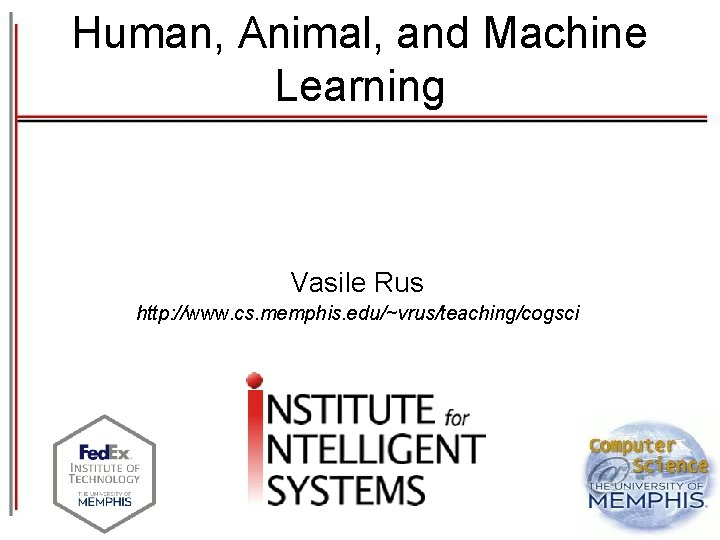Human, Animal, and Machine Learning Vasile Rus http: //www. cs. memphis. edu/~vrus/teaching/cogsci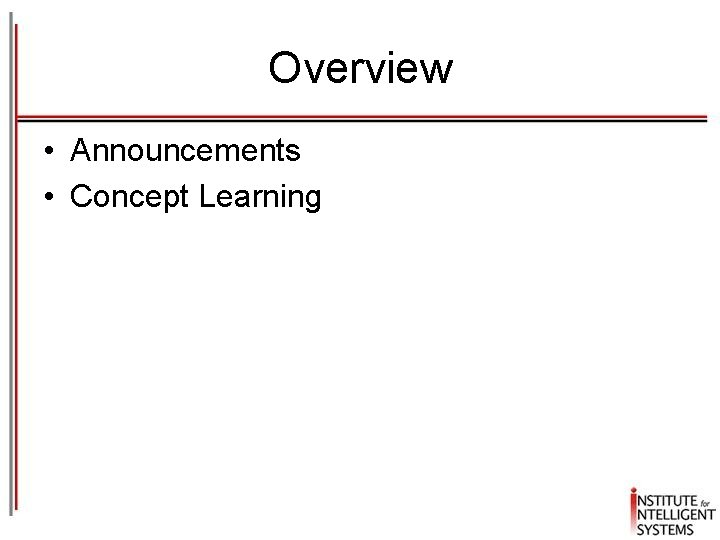Overview • Announcements • Concept LearningAnnouncements • Project Proposals – Due by Feb 12 • Assignment #2 – Frame your project in a ML problem using the framework discussed in week 1 [see Chapter 1 in the textbook]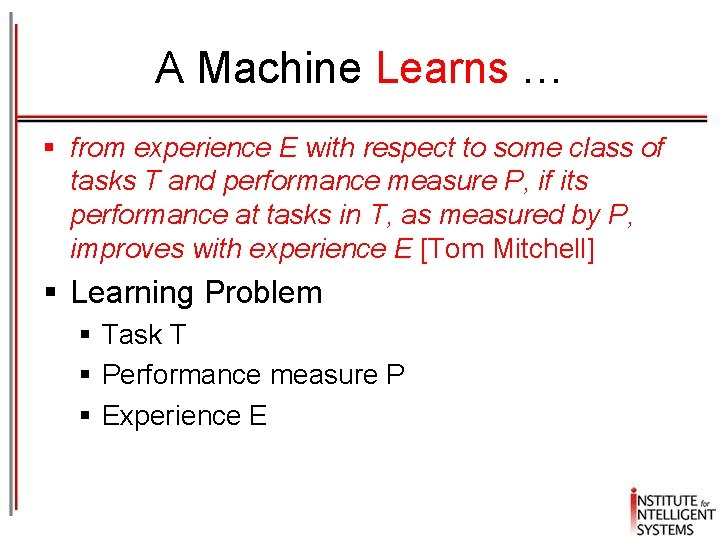A Machine Learns … § from experience E with respect to some class of tasks T and performance measure P, if its performance at tasks in T, as measured by P, improves with experience E [Tom Mitchell] § Learning Problem § Task T § Performance measure P § Experience E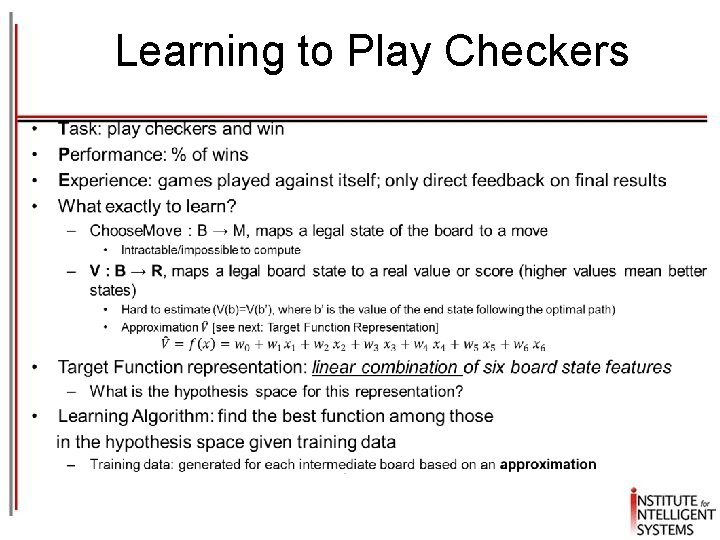Learning to Play Checkers •Concept Learning • Concept Learning algorithms • Hypothesis Space • Inductive BiasWhat is a Concept? • • “bird” “car” “attend the ML seminar” A subset of a larger set of “things/entities” that satisfy a certain property • Operational Definition – Inferring a Boolean-valued function from training examples of its input and output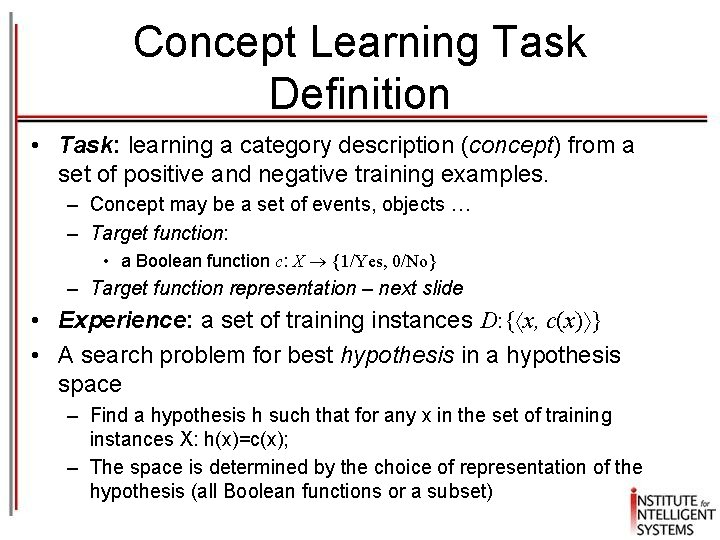Concept Learning Task Definition • Task: learning a category description (concept) from a set of positive and negative training examples. – Concept may be a set of events, objects … – Target function: • a Boolean function c: X {1/Yes, 0/No} – Target function representation – next slide • Experience: a set of training instances D: { x, c(x) } • A search problem for best hypothesis in a hypothesis space – Find a hypothesis h such that for any x in the set of training instances X: h(x)=c(x); – The space is determined by the choice of representation of the hypothesis (all Boolean functions or a subset)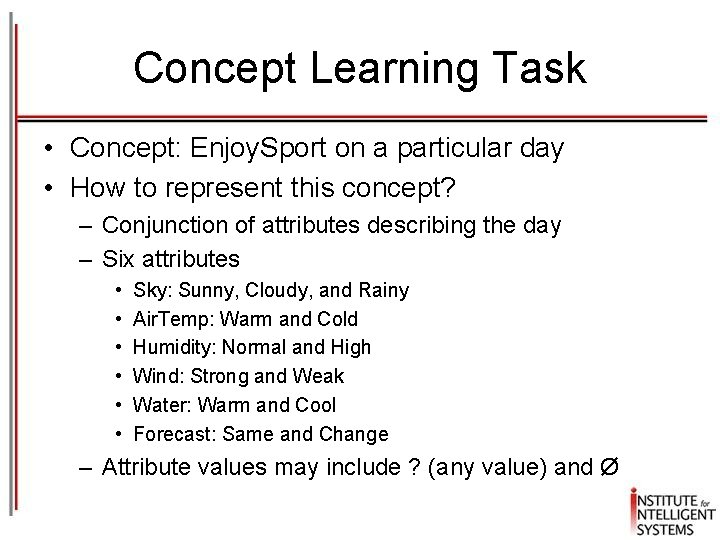Concept Learning Task • Concept: Enjoy. Sport on a particular day • How to represent this concept? – Conjunction of attributes describing the day – Six attributes • • • Sky: Sunny, Cloudy, and Rainy Air. Temp: Warm and Cold Humidity: Normal and High Wind: Strong and Weak Water: Warm and Cool Forecast: Same and Change – Attribute values may include ? (any value) and Ø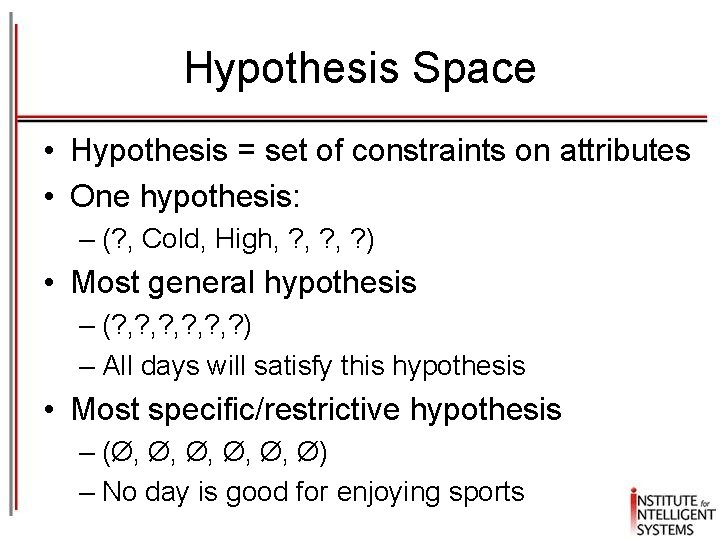Hypothesis Space • Hypothesis = set of constraints on attributes • One hypothesis: – (? , Cold, High, ? , ? ) • Most general hypothesis – (? , ? , ? , ? ) – All days will satisfy this hypothesis • Most specific/restrictive hypothesis – (Ø, Ø, Ø, Ø) – No day is good for enjoying sportsInstance and Hypothesis Space • Instance space X – 3 * 2 * 2 * 2 =96 distinct instances • Hypothesis space H – 5 * 4 * 4 * 4 = 5, 120 syntactically distinct hypotheses – 1 + (4 * 3 * 3 * 3) = 973 semantically unique hypotheses given that all hypotheses that contain a Ø are equivalent • Learning: search in the hypothesis space the hypothesis such that h(x)=c(x) for all x in the training data D (note that we do not say X anymore)Inductive Learning Hypothesis • Any hypothesis that approximates the target concept well over a large number of training instances will approximate well the target function well over other new, unobserved examplesDeduction vs. Induction Deduction Induction All humans are mortals. (Rule) Socrates is a human. (Fact) Socrates is human. Socrates is mortal. Conclusion: Socrates is a mortal. Generalization: All humans are mortal. D=training set We can at best guarantee that the output hypothesis fits the target concept over the training data X= all instances“Grue” Paradox • For each found hypothesis there an infinite number of inconsistent hypotheses (with the former) and which are consistent with the training data • One hypothesis derived from observing emeralds: All emeralds are green • Alternative, rival hypothesis: All emeralds are grue [i. e. green before year 2100 and blue afterwards] • Why should we prefer the former to the latter?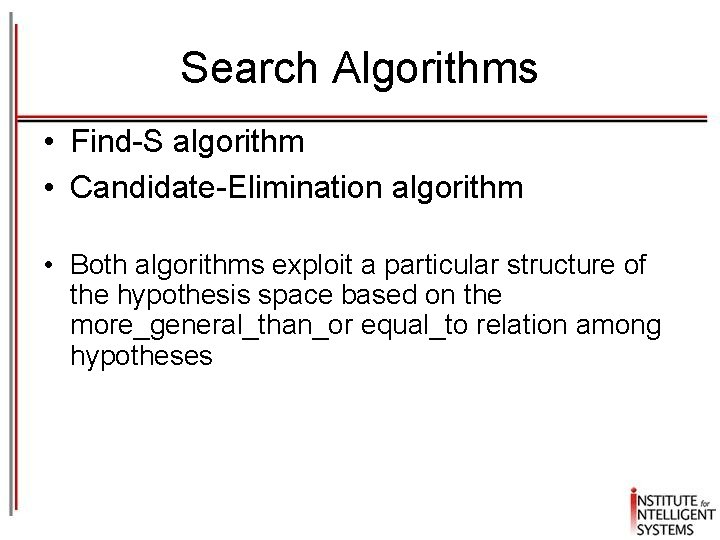Search Algorithms • Find-S algorithm • Candidate-Elimination algorithm • Both algorithms exploit a particular structure of the hypothesis space based on the more_general_than_or equal_to relation among hypotheses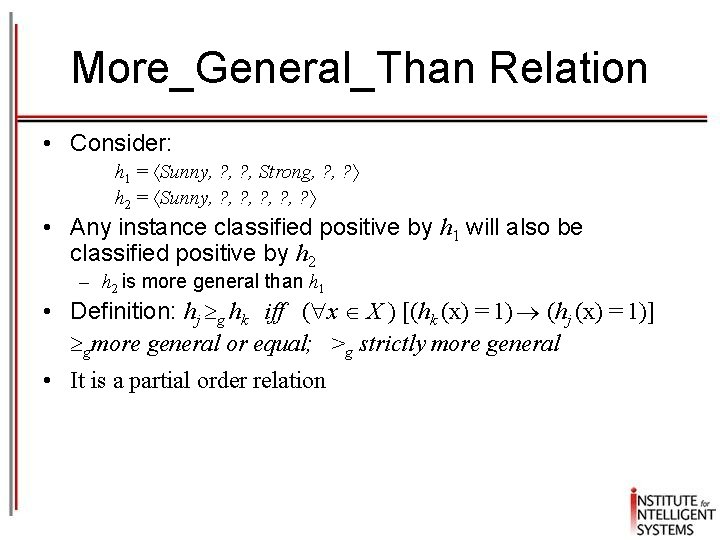More_General_Than Relation • Consider: h 1 = Sunny, ? , Strong, ? h 2 = Sunny, ? , ? , ? • Any instance classified positive by h 1 will also be classified positive by h 2 – h 2 is more general than h 1 • Definition: hj g hk iff ( x X ) [(hk (x) = 1) (hj (x) = 1)] gmore general or equal; >g strictly more general • It is a partial order relation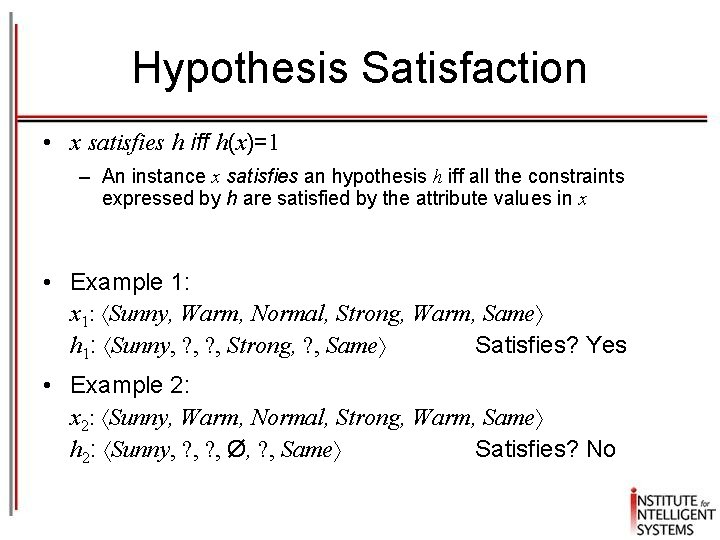Hypothesis Satisfaction • x satisfies h iff h(x)=1 – An instance x satisfies an hypothesis h iff all the constraints expressed by h are satisfied by the attribute values in x • Example 1: x 1: Sunny, Warm, Normal, Strong, Warm, Same h 1: Sunny, ? , Strong, ? , Same Satisfies? Yes • Example 2: x 2: Sunny, Warm, Normal, Strong, Warm, Same h 2: Sunny, ? , Ø, ? , Same Satisfies? No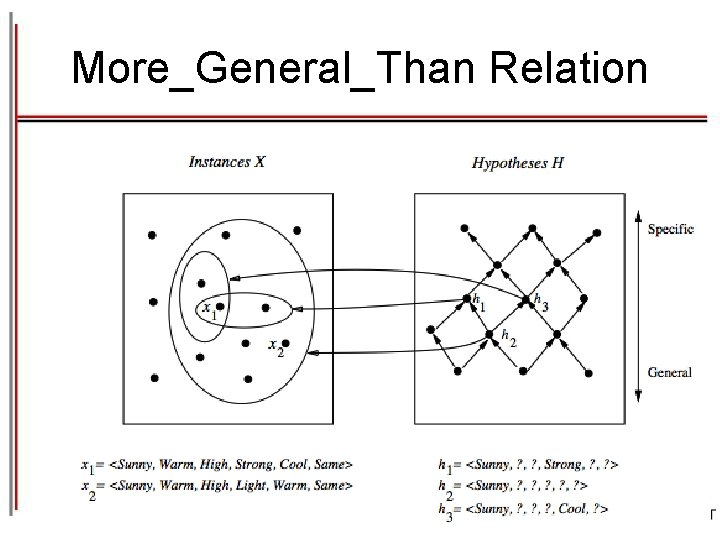More_General_Than RelationFormal task description • Given: – X all possible days, as described by the attributes – A set of hypothesis H, a conjunction of constraints on the attributes, representing a function h: X {0, 1} [h(x) = 1 if x satisfies h; h(x) = 0 if x does not satisfy h] – A target concept: c: X {0, 1} where c(x) = 1 iff Enjoy. Sport = Yes; c(x) = 0 iff Enjoy. Sport = No; – A training set of possible instances D: { x, c(x) } • Goal: find a hypothesis h in H such that h(x) = c(x) for all x in X Hopefully h will be able to predict outside D…The inductive learning assumption § We can at best guarantee that the output hypothesis fits the target concept over the training data § Assumption: an hypothesis that approximates well the training data will also approximate the target function over unobserved examples § i. e. given a significant training set, the output hypothesis is able to make predictionsFind-S: finding the most specific hypothesis 1. Initialize h to the most specific hypothesis in H 2. For each positive training instance: for each attribute constraint ai in h: If the constraint ai is satisfied by x then do nothing else replace ai in h by the next more general constraint satisfied by x (generalize) 3. Output hypothesis hFind-S in action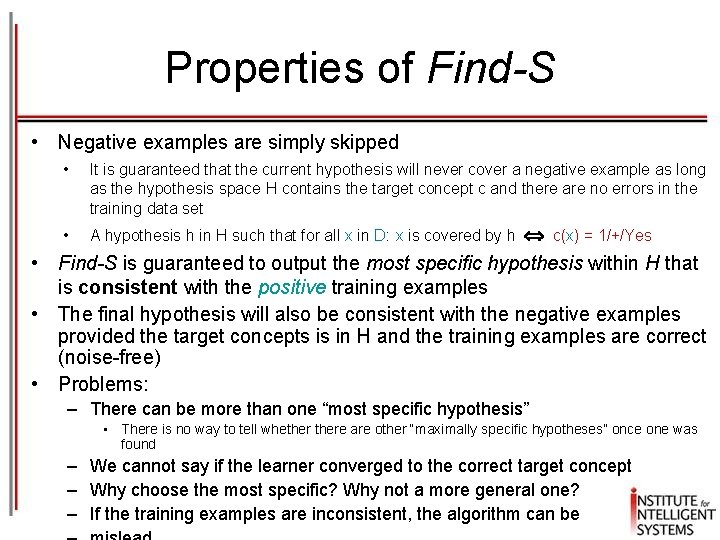Properties of Find-S • Negative examples are simply skipped • It is guaranteed that the current hypothesis will never cover a negative example as long as the hypothesis space H contains the target concept c and there are no errors in the training data set • A hypothesis h A hypothesis in H in such that for all x such that for all in D in : x is covered by h is covered by c(x) = 1/+/Yes • Find-S is guaranteed to output the most specific hypothesis within H that is consistent with the positive training examples • The final hypothesis will also be consistent with the negative examples provided the target concepts is in H and the training examples are correct (noise-free) • Problems: – There can be more than one “most specific hypothesis” • There is no way to tell whethere are other “maximally specific hypotheses” once one was found – We cannot say if the learner converged to the correct target concept – Why choose the most specific? Why not a more general one? – If the training examples are inconsistent, the algorithm can beCandidate elimination algorithm: the idea § The idea: output a description of the set of all hypotheses consistent with the training examples. § A hypothesis h is consistent with a set of training examples D if and only if h(x)=c(x) for each example (x, c(x)) in D. § Version space: a representation of the set of hypotheses which are consistent with D Consistent(h, D) ( x, c(x) D) h(x) = c(x)) 1. an explicit list of hypotheses (List-Then-Eliminate) 2. a compact representation of hypotheses which exploits the more_general_than partial ordering (Candidate-Elimination)Version space • The version space VSH, D is the subset of hypotheses from H consistent with the training example in D VSH, D {h H | Consistent(h, D)} Note: "x satisfies h" (h(x)=1) different from “h consistent with x" When an hypothesis h is consistent with a negative example d = x, c(x)=No , then x must not satisfy hThe List-Then-Eliminate algorithm Version space as list of hypotheses 1. Version. Space a list containing every hypothesis in H 2. For each training example, x, c(x) Remove from Version. Space any hypothesis h for which h(x) c(x) 3. Output the list of hypotheses in Version. Space • Pros: –it guarantees the return of all consistent hypotheses • Cons: –the hypothesis space must be finite as enumeration of all the hypotheses is not possible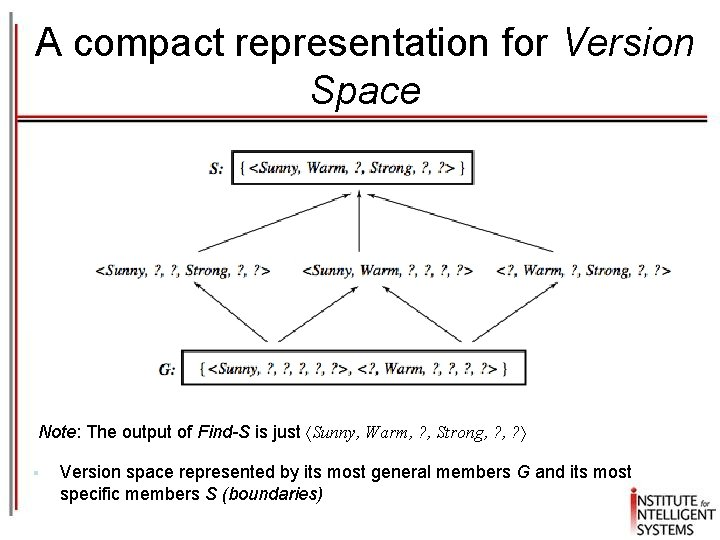A compact representation for Version Space Note: The output of Find-S is just Sunny, Warm, ? , Strong, ? § Version space represented by its most general members G and its most specific members S (boundaries)General and specific boundaries § The Specific boundary, S, of version space VSH, D is the set of its minimally general (most specific) members S {s H | Consistent(s, D) ( s' H)[(s gs') Consistent(s', D)]} Note: any member of S is satisfied by all positive examples, but more specific hypotheses fail to capture some § The General boundary, G, of version space VSH, D is the set of its maximally general members G {g H | Consistent(g, D) ( g' H)[(g' g g) Consistent(g', D)]} Note: any member of G is satisfied by no negative example but more general hypothesis cover some negative example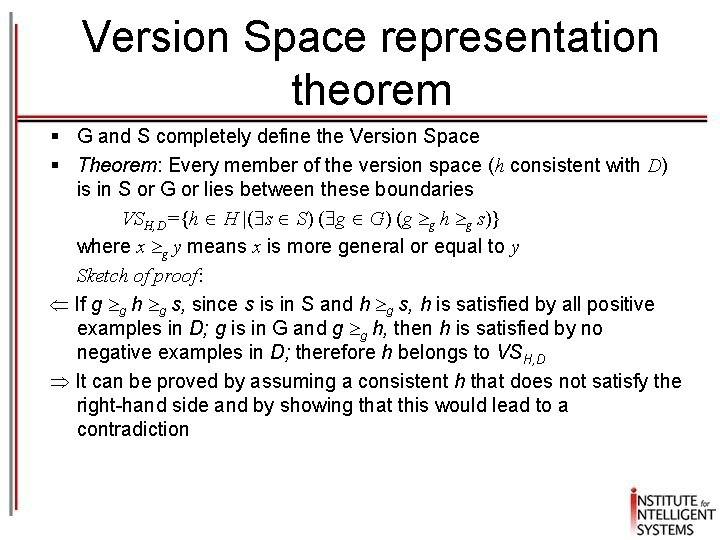Version Space representation theorem § G and S completely define the Version Space § Theorem: Every member of the version space (h consistent with D) is in S or G or lies between these boundaries VSH, D={h H |( s S) ( g G) (g g h g s)} where x g y means x is more general or equal to y Sketch of proof: If g g h g s, since s is in S and h g s, h is satisfied by all positive examples in D; g is in G and g g h, then h is satisfied by no negative examples in D; therefore h belongs to VSH, D It can be proved by assuming a consistent h that does not satisfy the right-hand side and by showing that this would lead to a contradiction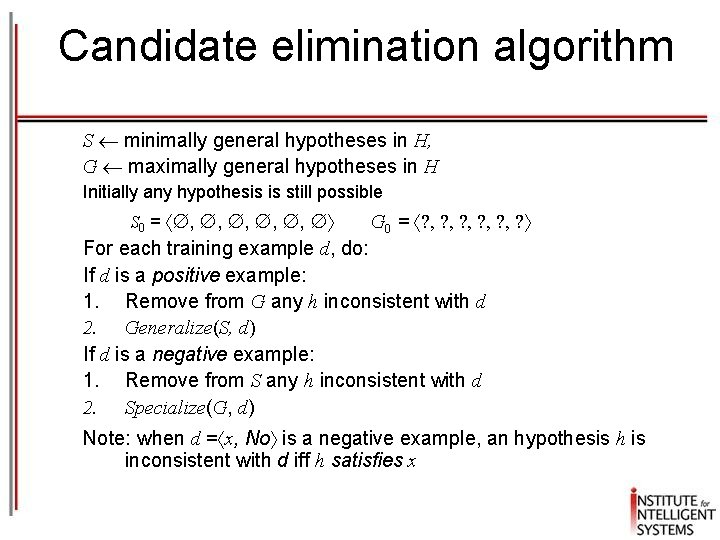Candidate elimination algorithm S minimally general hypotheses in H, G maximally general hypotheses in H Initially any hypothesis is still possible S 0 = , , , G 0 = ? , ? , ? , ? For each training example d, do: If d is a positive example: 1. Remove from G any h inconsistent with d 2. Generalize(S, d) If d is a negative example: 1. Remove from S any h inconsistent with d 2. Specialize(G, d) Note: when d = x, No is a negative example, an hypothesis h is inconsistent with d iff h satisfies xCandidate elimination algorithm Generalize(S, d): d is positive For each hypothesis s in S not consistent with d: 1. Remove s from S 2. Add to S all minimal generalizations of s consistent with d and having a generalization in G 3. Remove from S any hypothesis that is more general than a more specific h in S Specialize(G, d): d is negative For each hypothesis g in G not consistent with d: i. e. g satisfies d, but d is negative 1. Remove g from G 2. Add to G all minimal specializations of g consistent with d and having a specialization in S 3. Remove from G any hypothesis that is more specific than another hypothesis in G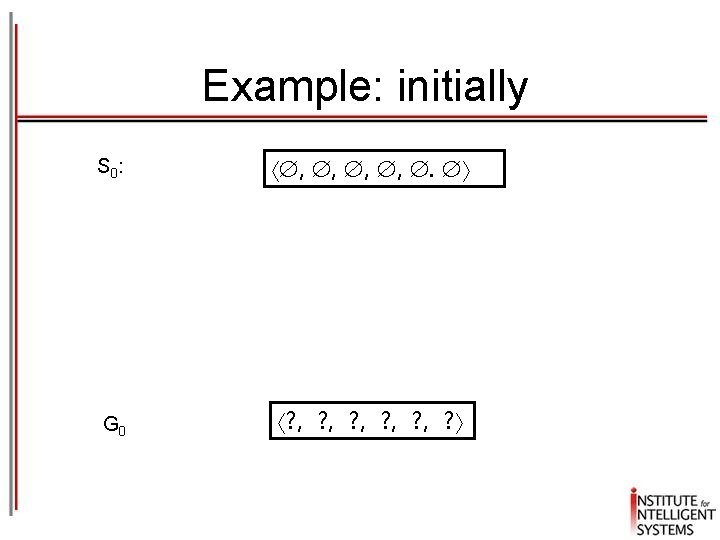Example: initially S 0 : , , . G 0 ? , ? , ? , ?Example: after Sunny, Warm, Normal, Strong, Warm, Same + S 0 : S 1 : G 0, G 1 , , . Sunny, Warm, Normal, Strong, Warm, Same ? , ? , ? , ?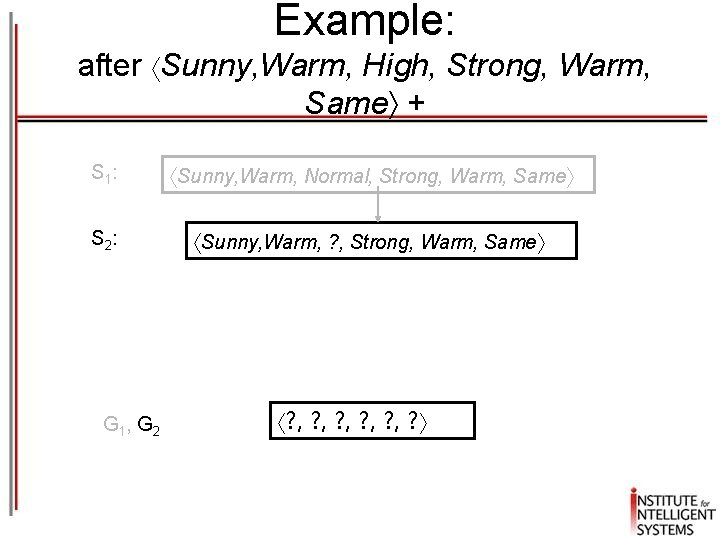Example: after Sunny, Warm, High, Strong, Warm, Same + S 1 : Sunny, Warm, Normal, Strong, Warm, Same S 2 : Sunny, Warm, ? , Strong, Warm, Same G 1, G 2 ? , ? , ? , ?Example: after Rainy, Cold, High, Strong, Warm, Change S 2, S 3: G 3 : Sunny, Warm, ? , Strong, Warm, Same Sunny, ? , ? , ? ? , Warm, ? , ? , ? , Same G 2 : ? , ? , ? , ?Example: after Sunny, Warm, High, Strong, Cool Change + S 3 S 4 G 4 : G 3 : Sunny, Warm, ? , Strong, Warm, Same Sunny, Warm, ? , Strong, ? Sunny, ? , ? , ? ? , Warm, ? , ? ? , ? , ? , Same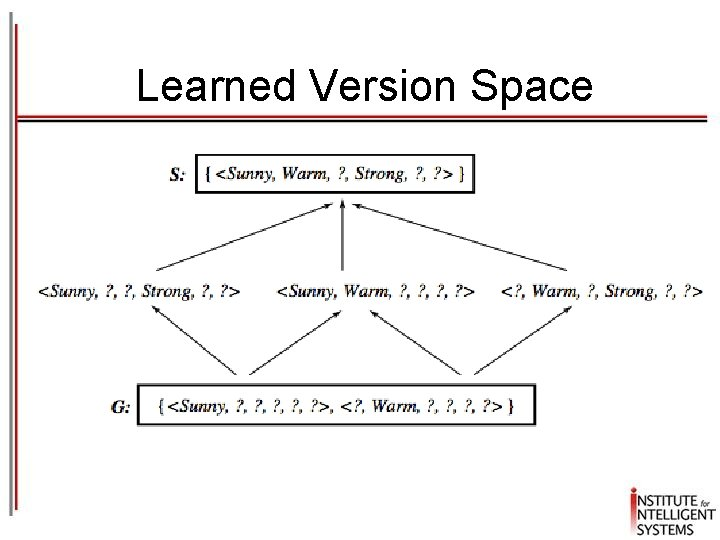Learned Version SpaceObservations § The learned Version Space correctly describes the target concept, provided: 1. There are no errors in the training examples 2. There is some hypothesis that correctly describes the target concept § § § If S and G converge to a single hypothesis the concept is exactly learned In case of errors in the training, useful hypothesis are discarded, no recovery possible An empty version space means no hypothesis in H is consistent with training examplesOrdering on training examples § The learned version space does not change with different orderings of training examples § Efficiency does § Optimal strategy (if you are allowed to choose) § Generate instances that satisfy half the hypotheses in the current version space. For example: Sunny, Warm, Normal, Light, Warm, Same satisfies 3/6 hyp. § Ideally the VS can be reduced by half at each experiment § Correct target found in log 2|VS| experimentsUse of partially learned concepts Classified as positive by all hypotheses, since satisfies any hypothesis in S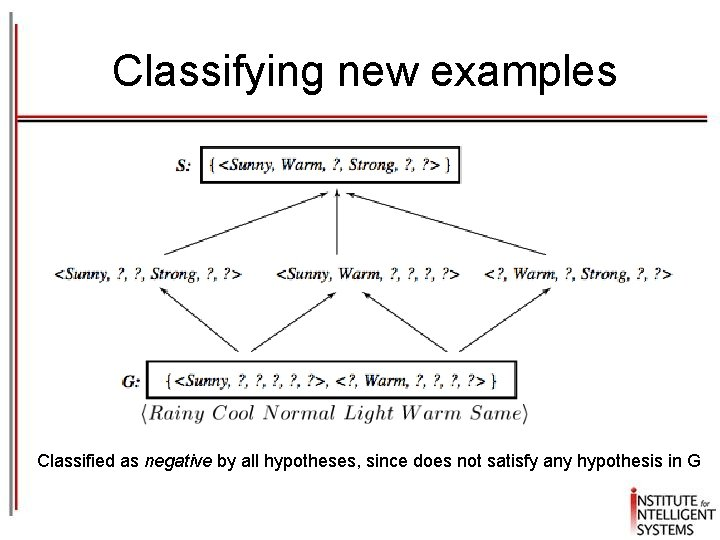Classifying new examples Classified as negative by all hypotheses, since does not satisfy any hypothesis in G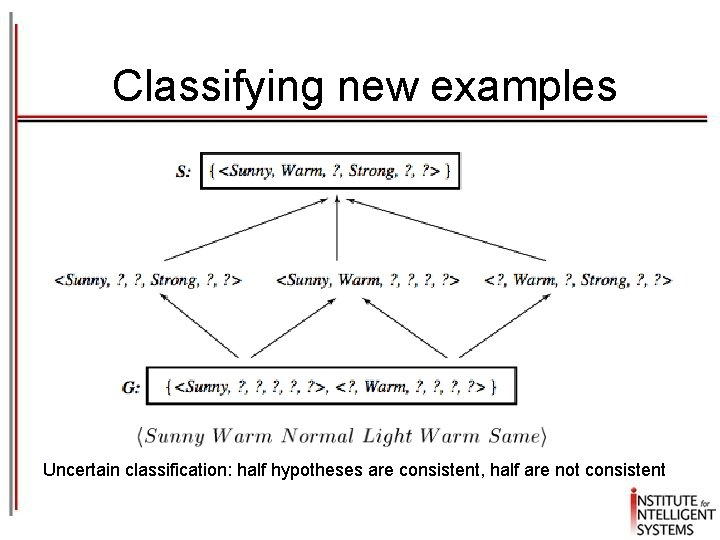Classifying new examples Uncertain classification: half hypotheses are consistent, half are not consistent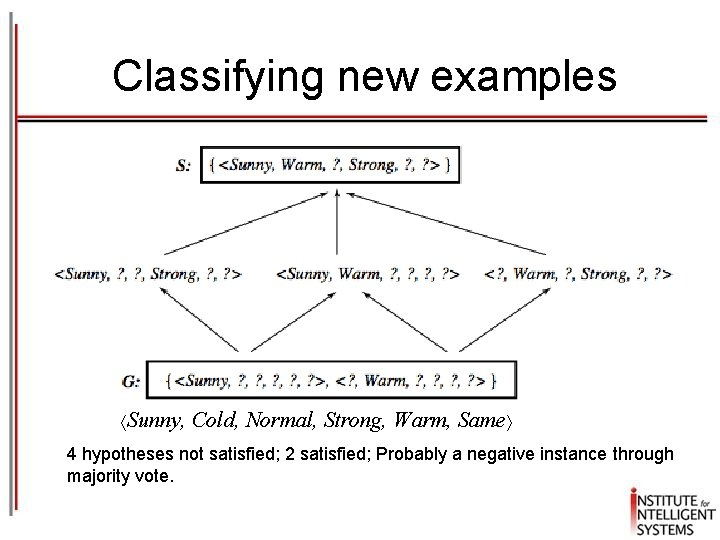Classifying new examples Sunny, Cold, Normal, Strong, Warm, Same 4 hypotheses not satisfied; 2 satisfied; Probably a negative instance through majority vote.Questions • What if H does not contain the target concept? – Can we improve the situation by expanding the hypothesis space? – Will the size of the hypothesis space influence the ability to generalize? – How many training examples are needed for a particular size of the hypothesis space? • These are general questions for inductive inference which we address in the context of Candidate-Elimination • Suppose we include in H every possible hypothesis … including the ability to represent disjunctive concepts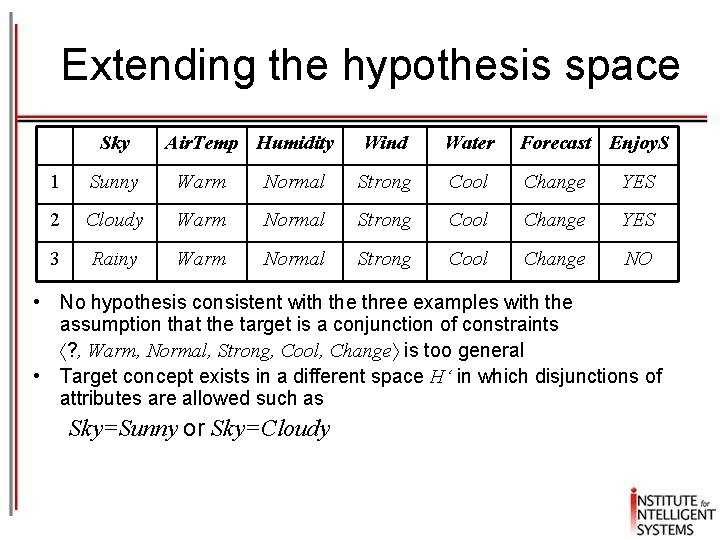Extending the hypothesis space Sky Air. Temp Humidity Wind Water Forecast Enjoy. S 1 Sunny Warm Normal Strong Cool Change YES 2 Cloudy Warm Normal Strong Cool Change YES 3 Rainy Warm Normal Strong Cool Change NO • No hypothesis consistent with the three examples with the assumption that the target is a conjunction of constraints ? , Warm, Normal, Strong, Cool, Change is too general • Target concept exists in a different space H‘ in which disjunctions of attributes are allowed such as Sky=Sunny or Sky=Cloudy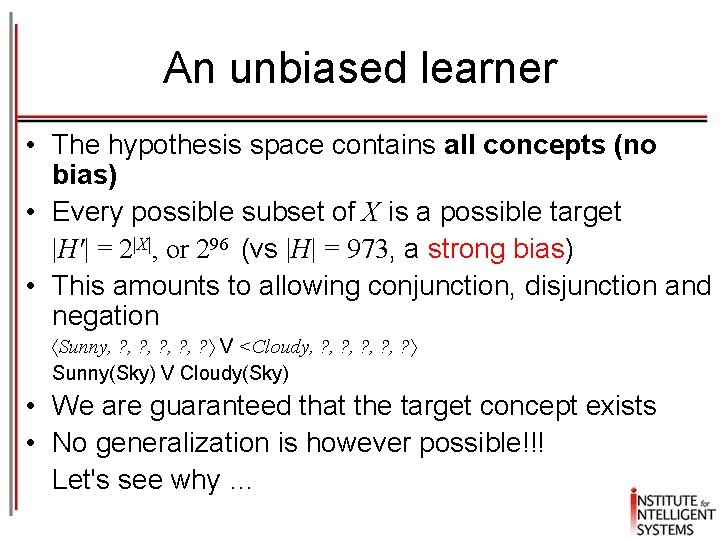An unbiased learner • The hypothesis space contains all concepts (no bias) • Every possible subset of X is a possible target |H'| = 2|X|, or 296 (vs |H| = 973, a strong bias) • This amounts to allowing conjunction, disjunction and negation Sunny, ? , ? , ? V <Cloudy, ? , ? , ? Sunny(Sky) V Cloudy(Sky) • We are guaranteed that the target concept exists • No generalization is however possible!!! Let's see why …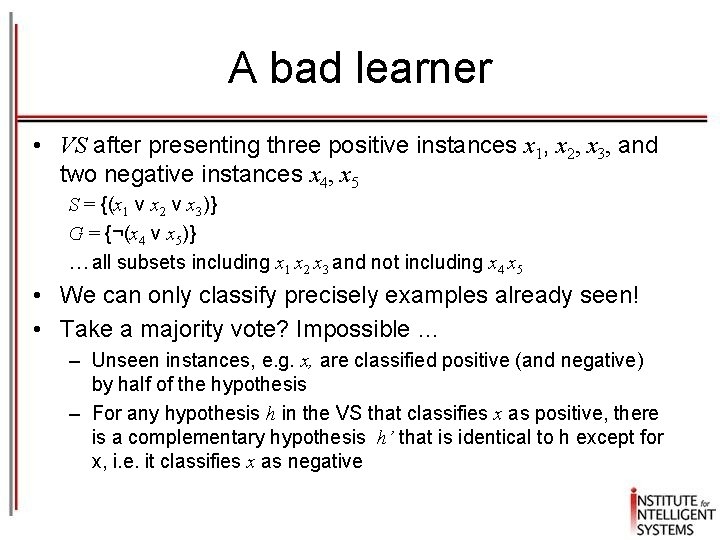A bad learner • VS after presenting three positive instances x 1, x 2, x 3, and two negative instances x 4, x 5 S = {(x 1 v x 2 v x 3)} G = {¬(x 4 v x 5)} … all subsets including x 1 x 2 x 3 and not including x 4 x 5 • We can only classify precisely examples already seen! • Take a majority vote? Impossible … – Unseen instances, e. g. x, are classified positive (and negative) by half of the hypothesis – For any hypothesis h in the VS that classifies x as positive, there is a complementary hypothesis h’ that is identical to h except for x, i. e. it classifies x as negative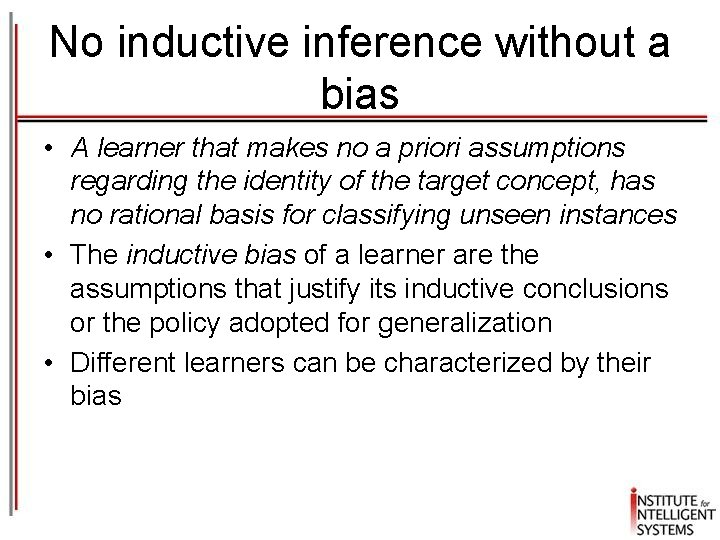No inductive inference without a bias • A learner that makes no a priori assumptions regarding the identity of the target concept, has no rational basis for classifying unseen instances • The inductive bias of a learner are the assumptions that justify its inductive conclusions or the policy adopted for generalization • Different learners can be characterized by their bias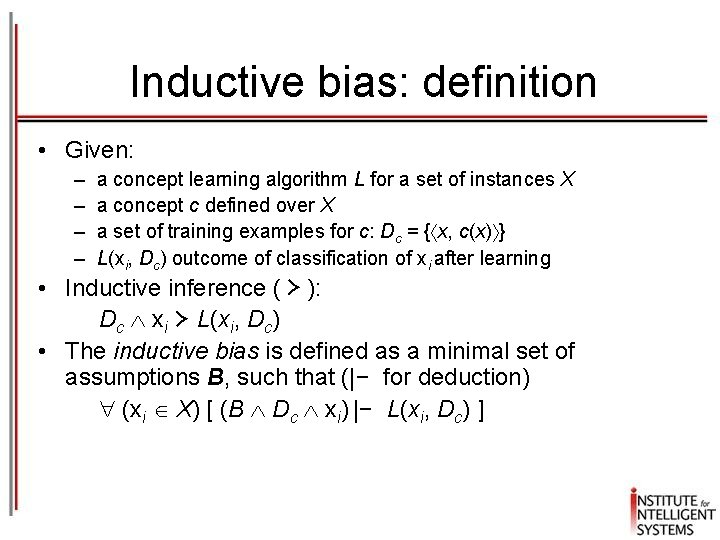Inductive bias: definition • Given: – – a concept learning algorithm L for a set of instances X a concept c defined over X a set of training examples for c: Dc = { x, c(x) } L(xi, Dc) outcome of classification of xi after learning • Inductive inference ( ≻ ): Dc xi ≻ L(xi, Dc) • The inductive bias is defined as a minimal set of assumptions B, such that (|− for deduction) (xi X) [ (B Dc xi) |− L(xi, Dc) ]Inductive bias of Candidate. Elimination § Assume L is defined as follows: § § compute VSH, D classify new instance by complete agreement of all the hypotheses in VSH, D Then the inductive bias of Candidate-Elimination is simply B (c H) In fact by assuming c H: 1. c VSH, D , because VSH, D includes all hypotheses in H consistent with D 2. L(xi, Dc) outputs a classification "by complete agreement", hence any hypothesis, including c, outputs L(xi, Dc)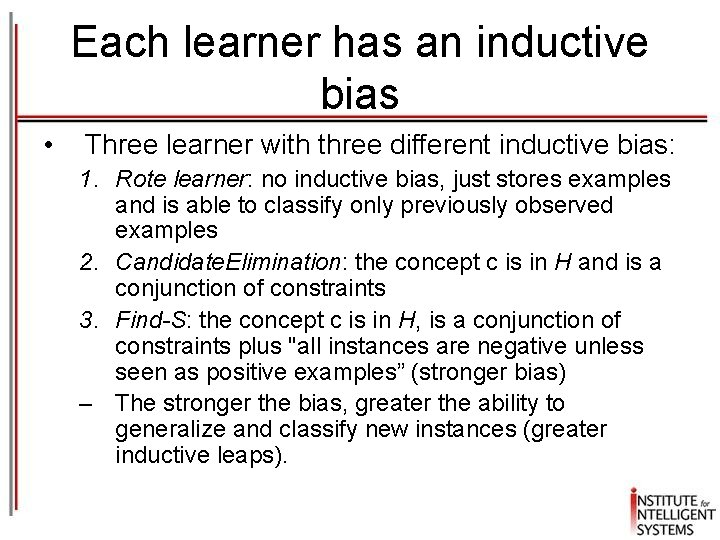Each learner has an inductive bias • Three learner with three different inductive bias: 1. Rote learner: no inductive bias, just stores examples and is able to classify only previously observed examples 2. Candidate. Elimination: the concept c is in H and is a conjunction of constraints 3. Find-S: the concept c is in H, is a conjunction of constraints plus "all instances are negative unless seen as positive examples” (stronger bias) – The stronger the bias, greater the ability to generalize and classify new instances (greater inductive leaps).Summary • Concept LearningNext Time • Decision Trees